# Bond Length

Bond length is defined as the equilibrium distance between the nuclei of two bonded atoms in a molecule.

Bond length increases with increases is the size of bonded atoms and decreases with an increase in the number of bonds between bonded atoms.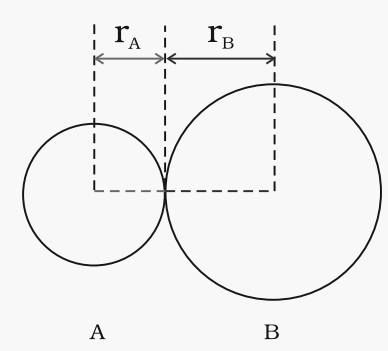Fig. The bond length in a covalent molecule AB.

R = rA + rB (R is the bond length and rA and rB are the covalent radii of atoms A and B respectively)

# Bond Angle

In a covalently bonded molecule having more than two atoms, the bonds form an angle with each other, which known as bond angle. In general, an increase in the size of central atom decreases the bond angle.

Factors affecting bond angle:

• Lone pair repulsion
• Hybridisation of central room.

It is determined by X-rays diffraction method.

For example: H-O-H bond angle in water can be represented as under: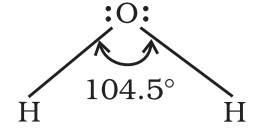# Bond Enthalpy

It is defined as the amount of energy required to break one mole of bonds of a particular type to separate them into gaseous atoms.

Bond enthalpy is also known as bond dissociation enthalpy or simply enthalpy.

Unit of bond enthalpy = kJ mol-1.

Greater the bond enthalpy, stronger is the bond. For e.g., the H-H bond enthalpy in hydrogen is 435.8 kJ mol-1.

The magnitude of bond enthalpy is also related to bond multiplicity. Greater the bond multiplicity, more will be the bond enthalpy. For e.g., bond enthalpy of C-C bond is 347 kJ mol-1 while that of C=C bond is 610 kJ mol-1.

In polyatomic molecules, the term mean or average bond enthalpy is used.# Bond Order

According to Lewis, in a covalent bond, the bond order is given by the number of bonds between two atoms in a molecule. For example,

Bond order of H2 (H-H) = 1

Bond order of O2 (O=O) = 2

Bond order of N2 (N=N) = 3

Isoelectronic molecules and ions have identical bond orders. For example, F2 and O22- have bond order = 1.

N2, CO and NO+ have bond order = 3.

With the increase in bond order, bond enthalpy increases and bond length decreases. For example,

For N2, Bond order = 3

Bond enthalpy = 946 kJ/mol.

For O2, Bond order = 2

Bond enthalpy = 498 kJ/mol.

# Resonance Structures

According to the concept of resonance, whenever a single Lewis structure cannot describe a molecule accurately, a number of structures with similar energy, positions of nuclei, bonding and non-bonding pairs of electrons are taken as the canonical structures of the hybrid which describes the molecule accurately.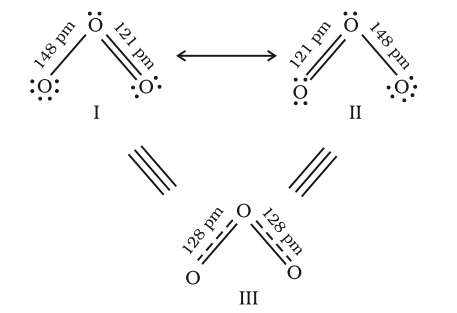Fig. Resonance in the O3 molecule
(structures I and II represent the two canonical forms while the structure III is the resonance hybrid)

# Polarity of Bonds

## Non-Polar Covalent Bonds:

When the atoms joined by covalent bond are the same like; H2, O2, Cl2, the shared pair of electrons is equally attracted by two atoms and thus the shared electron pair is equidistant to both of them.

Alternatively, we can say that it lies exactly in the centre of the bonding atoms. As a result, no poles are developed and the bond is called as non-polar covalent bond. The corresponding molecules are known as non-polar molecules.

For example,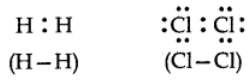## Polar bond:

When covalent bonds formed between different atoms of different electronegativity, shared electron pair between two atom gets displaced towards highly electronegative atoms.

Greater the difference in electronegativity of the bonding atoms, more will be polarity of the bond.# Dipole Moment

The product of the magnitude of the charge and the distance between the charge of positive and negative charges.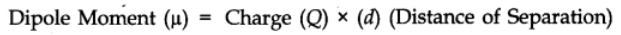It is a vector quantity and is represented by an arrow with its tail at the positive charge and head pointing towards a negative charge.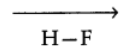With the help of diploe moment, the degree of polarity of bonds can be expressed.

It is expressed in Debye units (D)

1D = 3.33564 x 10-30 cm

### Application of Dipole Moment

• For determining the polarity of the molecules.
• In finding the shapes of the molecules.

For example, the molecules with zero diploe moment will be linear or symmetrical. Those molecules which have unsymmetrical shapes will be either bent or angular.
(e.g., NH3 with μ = 1.47 D).

• In calculating the percentage ionic character of polar bonds.